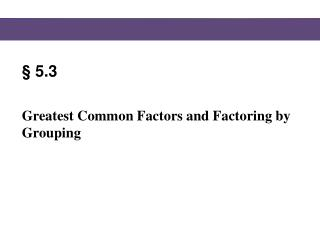DownloadDownload Presentation§ 5.3

# § 5.3

Download Presentation## § 5.3

- - - - - - - - - - - - - - - - - - - - - - - - - - - E N D - - - - - - - - - - - - - - - - - - - - - - - - - - -
##### Presentation Transcript

1. §5.3 Greatest Common Factors and Factoring by Grouping

2. Factoring Factoring a polynomial means finding an equivalent expression that is a product. For example, when we take the polynomial And write it as we say that we have factored the polynomial. In factoring, we write a sum as a product. Blitzer, Intermediate Algebra, 5e – Slide #2 Section 5.3

3. Factoring Blitzer, Intermediate Algebra, 5e – Slide #3 Section 5.3

4. Factoring EXAMPLE Factor: SOLUTION First, we determine the greatest common factor of the three terms. Notice that the greatest integer that divides into 49, 70 and 35 (the coefficients of the terms) is 7. The variables raised to the smallest exponents are The GCF is P 332 Blitzer, Intermediate Algebra, 5e – Slide #4 Section 5.3

5. Factoring CONTINUED Express each term as the product of the GCF and its other factor Factor out the GCF P 332 Blitzer, Intermediate Algebra, 5e – Slide #5 Section 5.3

6. A Strategy for Factoring Polynomials, page 363 /////////////////////////////////////////// Blitzer, Intermediate Algebra, 5e – Slide #6 Section 5.6

7. Factoring EXAMPLE P 333 Factor: SOLUTION The GCF is 5x. Because the leading coefficient, -5, is negative, we factor out a common factor with a negative coefficient. We will factor out the negative of the GCF, or -5x. Express each term as the product of the GCF and its other factor Important to factor the negative sign Factor out the GCF Blitzer, Intermediate Algebra, 5e – Slide #7 Section 5.3

8. Factoring Check Point 1 Factor Check Point 2a Factor P 332-333 Blitzer, Intermediate Algebra, 5e – Slide #8 Section 5.2

9. Factoring Check Point 2b Factor Check Point 2c Factor P 332-333 Blitzer, Intermediate Algebra, 5e – Slide #9 Section 5.2

10. Factoring Check Point 3 Factor Want a to be positive P 334 Blitzer, Intermediate Algebra, 5e – Slide #10 Section 5.2

11. Factoring by Grouping EXAMPLE Factor: SOLUTION Let’s identify the common binomial factor in each part of the problem. The GCF, a binomial, is x + y. The GCF, a binomial, is x + y. P 334 Blitzer, Intermediate Algebra, 5e – Slide #11 Section 5.3

12. Factoring by Grouping CONTINUED We factor out the common binomial factor as follows. This step, usually omitted, shows each term as the product of the GCF and its other factor, in that order. Factor out the GCF Blitzer, Intermediate Algebra, 5e – Slide #12 Section 5.3

13. Factoring Check Point 4a Factor Check Point 4b Factor P 334 Blitzer, Intermediate Algebra, 5e – Slide #13 Section 5.2

14. Factoring by Grouping P 334 Blitzer, Intermediate Algebra, 5e – Slide #14 Section 5.3

15. Factoring by Grouping EXAMPLE P 334 Factor: SOLUTION There is no factor other than 1 common to all terms. However, we can group terms that have a common factor: + Common factor is : Use -2b, rather than 2b, as the common factor: -2bx – 4by = -2b(x + 2y). In this way, the common binomial factor, x + 2y, appears. Blitzer, Intermediate Algebra, 5e – Slide #15 Section 5.3

16. Factoring by Grouping CONTINUED P 334 The voice balloons illustrate that it is sometimes necessary to use a factor with a negative coefficient to obtain a common binomial factor for the two groupings. We now factor the given polynomial as follows: Group terms with common factors Factor out the common factors from the grouped terms Factor out the GCF Blitzer, Intermediate Algebra, 5e – Slide #16 Section 5.3

17. Factoring Check Point 5 Factor Check Point 6 Factor P 335-6 Blitzer, Intermediate Algebra, 5e – Slide #17 Section 5.2

18. DONE

19. Factoring by Grouping EXAMPLE • Your local electronics store is having an end-of-the-year sale. The price on a large-screen television had been reduced by 30%. Now the sale price is reduced by another 30%. If x is the television’s original price, the sale price can be represented by • (x – 0.3x) – 0.3(x – 0.3x) • Factor out (x – 0.3x) from each term. Then simplify the resulting expression. • Use the simplified expression from part (a) to answer these questions. With a 30% reduction followed by a 30% reduction, is the television selling at 40% of its original price? If not, at what percentage of the original price is it selling? Blitzer, Intermediate Algebra, 5e – Slide #19 Section 5.3

20. Factoring by Grouping CONTINUED SOLUTION (a) (x – 0.3x) – 0.3(x – 0.3x) = 1(x – 0.3x) – 0.3(x – 0.3x) This step, usually omitted, shows each term as the product of the GCF and its other factor, in that order. = (x – 0.3x)(1 – 0.3) Factor out the GCF = (x – 0.3x)(0.7) Subtract = 0.7x – 0.21x Distribute = 0.49x Subtract Blitzer, Intermediate Algebra, 5e – Slide #20 Section 5.3

21. Factoring by Grouping CONTINUED (b) With a 30% reduction, followed by another 30% reduction, the expression that represents the reduced price of the television simplifies to 0.49x. Therefore, this series of price reductions effectively gives a new price for the television at 49% its original price, not 40%. Blitzer, Intermediate Algebra, 5e – Slide #21 Section 5.3